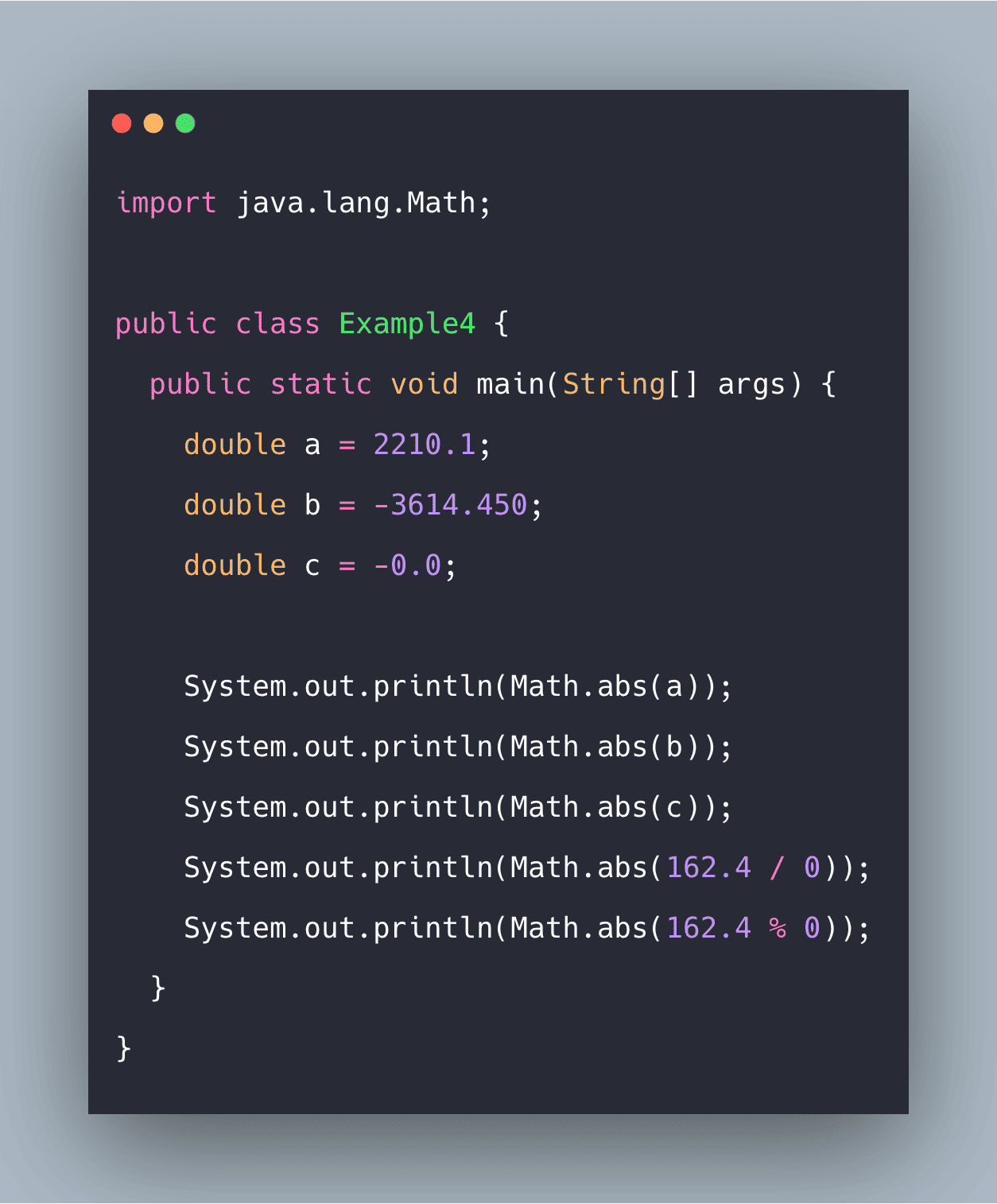# Java Math abs() Function ExampleJava Math abs() is an inbuilt function that returns the absolute (positive) value of an int value. The abs() method gives an absolute value of the argument.

To find the absolute value of a given argument in Java, the java.lang.Math.abs() method is used. This is often helpful when the program deals with mathematical expressions where the |x| value (the absolute value of a variable x) is required to be calculated.

### Java Math abs()

Java Math.abs() method returns the absolute (positive) value of an int value. The Math.abs() method gives the absolute value of the argument. The argument can be int, double, long, and float.

#### Syntax

``````public static int abs(int x)
public static long abs(long x)
public static float abs(float x)
public static double abs(double x)``````

## How to Install OpenJDK 11 on CentOS 8

What is OpenJDK? OpenJDk or Open Java Development Kit is a free, open-source framework of the Java Platform, Standard Edition (or Java SE).

## Math class in Java Example | Java Math Class Tutorial

Math class in Java Example. Using the Math class, we can implement methods like min(), max(), avg(), sin(), cos(), tan(), round(), ceil(), floor(), abs(), pow(), floor(), ciel(), round()

## Java Online Training | Java Online Course | ITGuru

Our Java Online Training provide you to learn about Java programming and its different features with realty. Our Java Online Course includes live sessions, live projects

## Best Java IDE 2020 | Most Popular Java IDE

Our Java Online Training provide you to learn about Java programming and its different features with realty. Our Java Online Course includes live sessions, live projects

## Learning by Doing: How to Learn Java Basics by Building Your Own Project

Check out some ideas for sample projects that can help you learn Java, and programming in general, in a fun and engaging way.